## Computer-Graphics

 Question 1

Which of the following statements is/are True regarding the solution to the visibility problem in 3D graphics ?

S1 : The Painter’s algorithm sorts polygons by depth and then paints (scan - converts) each Polygon onto the screen starting with the most nearest polygon. S2 : Backface Culling refers to eliminating geometry with back facing normals. Code :
 A S1 only B S2 only C Both S1 and S2 D Neither S1 Nor S2
Computer-Graphics       3D-Geometric-Transformation       UGC-NET CS 2018 JUNE Paper-2
Question 1 Explanation:
Visibility problem in 3D graphics
1. Painter's algorithm
- A depth sorting method
- Surfaces are sorted in the order of decreasing depth
- Surfaces are drawn in the sorted order, and overwrite the pixels in the frame buffer - Subtle difference from depth buffer approach: entire face drawn
- Two problems:
• It can be nontrivial to sort the surfaces
• There can be no solution for the sorting order
2. Back Face Culling
- Back faces: faces of opaque object which are “pointing away” from viewer
- Back face culling – remove back faces (supported by OpenGL)
How to detect back faces
- If we find backface, do not draw, save rendering resources
- There must be other forward face(s) closer to eye
- F is face of object we want to test if backface
- P is a point on F
- Form view vector, V as (eye – P)
- N is normal to face F
3. View-Frustum Culling
- Remove objects that are outside the viewing frustum
- Done by 3D clipping algorithm (e.g. Liang-Barsky)
4. Ray Tracing
- Ray tracing is another example of image space method
- Ray tracing: Cast a ray from eye through each pixel to the world
5. Z(Depth buffer algorithm)
Reference: https://web.cs.wpi.edu/~emmanuel/courses/cs543/slides/lecture08_p2.pdf
 Question 2
Memory mapped displays
 A are utilized for high resolution graphics such as maps B uses ordinary memory to store the display data in character form C stores the display data as individual bits D are associated with electromechanical teleprinters
Computer-Graphics       Display-System       Nielit Scientist-C 2016 march
Question 2 Explanation:
● Graphs can be displayed on a screen by writing character values into a special area of RAM within the video controller. ● Prior to cheap RAM that enabled bit-mapped displays, this character cell method was a popular technique for computer video displays.
 Question 3
While making bubbled lists, which of the following options are available?
 A Square,disc,tringle B Triangle,disc,circle C Triangle,square,circle D Disc,square,circle
Computer-Graphics       Shapes       KVS 22-12-2018 Part-B
Question 3 Explanation:
→ Disc: A filled circle
→ Circle : An unfilled circle
→ square : A filled square
 Question 4
MPEG involves both spatial compression and temporal compression. The spatial compression is similar to JPEG and temporal compression removes_____frames.
 A Voice B Spatial C Temporal D Redundant
Computer-Graphics       Motion-Pictures       KVS 22-12-2018 Part-B
Question 4 Explanation:
MPEG: The MPEG codec can only be used when the final video file will be in MPEG format (it is not compatible with other file types). It uses a lossy compression scheme (although it may be lossless at high-quality settings) and spatial and temporal compression. MPEG offers the best compression possible, but MPEGs are not yet as widely supported on the Web as other video formats.
Spatial compression:
Spatial (or intraframe) compression takes place on each individual frame of the video, compressing the pixel information as though it were a still image. JPEG, PNG and PICT files are an example of spatial compression.
It is known as the intraframe method.

Temporal compression:
It happens over a series of frames of the video. Temporal (or interframe) compression happens over a series of frames and takes advantage of areas of the image that remain unchanged from frame to frame, throwing out data for repeated pixels.
It is also called the interframe method.
 Question 5
In which of the following, the density of the core remains constant from the center to the edges?
 A Single mode fiber B Multimedia step-index fiber C Multimode graded index fiber D Single mode step index fiber
Computer-Graphics       Multimode-Step-Index       KVS 22-12-2018 Part-B
Question 5 Explanation:
Multimode Step-index: In multimode step-index fiber, the density of the core remains constant from the center to the edges. A beam of light moves through this constant density in a straight line until it reaches the interface of the core and the cladding
 Question 6
Which of the following is a special effect in motion pictures and animation that changes one image/picture into another through a seamless transition?
 A Modeling B Morphing C Animating D Wrapping
Computer-Graphics       Transformations       KVS 22-12-2018 Part-B
Question 6 Explanation:
→ Morphing is a special effect in motion pictures and animations that changes (or morphs) one image or shape into another through a seamless transition.
→ Morphing means stretching or as part of a fantasy or surreal sequence.
 Question 7
Even when the screen is completely dark while the film is in motion, commercial motion pictures use
 A 32 frames per second or 101 screen illuminations per second B 72 frames per second or 234 screen illuminations per second C 8 frames per second or 32 screen illuminations per second D 24 frames per second or 72 screen illuminations per second
Computer-Graphics       Motion-Pictures       KVS DEC-2013
Question 7 Explanation:
→ Motion picture, also called film or movie, series of still photographs on film, projected in rapid succession onto a screen by means of light.
→ When a motion picture film is projected on a screen at the rate of at least 16 illuminations per second but the commercial motion pictures uses 24 frames per second or 72 screen illuminations per second.
→ Film Technology standard is 24 frames per second, a three bladed shutter and some dreamy motion blur, all projected as shadow and light on the side of a wall.
 Question 8
The file size of a 640 by 480 pictures of 256 colours in a 8-bit resolution is
 A 300KB B 900KB C 128KB D 1024KB
Computer-Graphics       Pixel-Resolution       KVS DEC-2013
Question 8 Explanation:
●Give picture size with 640 by 480 resolution with 256 colors per pixel.
●This implies 640 * 480 = 307,200 pixels. Recall that 256 values requires 8 bits of computer storage.
●Hence (307,200 pixels) * (8 bits per pixel) = 2,457,600 bits of storage.
●A common method for selling computer storage is in 8 bit groupings called bytes.
●Hence the picture would require (2,457,600 bits) / (8 bits per byte) = 307,200 bytes of computer storage.
 Question 9
The process of producing bitmapped images from a view of 3-D models in a 3-D scene is called
 A Rendering B Looping C Cross dissolving D Imaging
Computer-Graphics       3D       KVS DEC-2013
Question 9 Explanation:
Rendering or image synthesis is the automatic process of generating a photorealistic or non-photorealistic image from a 2D or 3D model (or models in what collectively could be called a scene file) by means of computer programs.
 Question 10
Which of the following is interactive?
 A A radio broadcast B A talk show on TV C A newspaper D A computer game
Computer-Graphics       I/O-Devices       KVS DEC-2013
Question 10 Explanation:
Options A,B and C are not interactive , it will provide only information to the people. Where as Computer game in which computer user should interact with the computer in order to play the game
 Question 11
Anti aliasing is important to improve readability of text. It deals with the
 A Elimination of "jaggies" B Spacing between two individual characters C Underlining letters D Spacing of a group of characters
Computer-Graphics       Anti-Aliasing       KVS DEC-2017
Question 11 Explanation:
● Antialiasing removes jagged edges by adding subtle color changes around the lines, tricking the human eye into thinking that the lines are not jagged.
● The slight changes in color around the edges of an image help the line blend around curves, giving the impression that the line is true.
● These color changes are made on a very small scale that the human eye cannot detect under normal circumstances. In order to be able to see that an image has been antialiased, it would have to be magnified.
 Question 12
What is the bitrate for transmitting uncompressed 800x600 pixel color frame with 8 bits/pixel at 40 frames/Second?
 A 1536 Mbps B 2.4 Mbps C 15.36 Mbps D 153.6 Mbps
Computer-Graphics       Bit-Rate       KVS DEC-2017
Question 12 Explanation:
Step-1: Given data, Uncompressed pixel = 800*600
Step-2: Each of 8 bit = 800*600 *8
Step-3: Bit rate for transmitting = 800*600 *8 *40 =153600000 bits
Step-4: Here, they are given in Mbps. 153600000 bits =153.6Mbps
 Question 13
The easiest method in flash to draw a heptagon, is to use the___
 A Polystar tool with the "Polygon" style B Polygon Tool C Lasso Tool with the "create star" option D Polystar Tool with the "star" style
Computer-Graphics       Tools       KVS DEC-2017
Question 13 Explanation:
There are 3 tools for drawing geometric shapes
1. Rectangle(Rectangle tool)
2. Oval(Oval tool)
3. Polygon(Polystar tool)
Note: We are not using Option A,C and D for drawing heptagon.
 Question 14
___ is a special effect in motion pictures and animations that changes one image or sharp into another through a seamless transition
 A Tweening B Inverse kinematics C Morphing D Tweaking
Computer-Graphics       Transformations       KVS DEC-2017
Question 14 Explanation:
Morphing is a special effect in motion pictures and animations that changes (or morphs) one image or shape into another through a seamless transition. morphing means stretching or as part of a fantasy or surreal sequence. Traditionally such a depiction would be achieved through cross-fading techniques on film.
 Question 15
Given that a 22 inch monitor with an aspect ratio of 16:9 has a monitor of 1920x1080, what is the width of the monitor?
 A 22 inches B 8:53 inches C 10:79 inches D 19:17 inches
Computer-Graphics       Aspect-Ratio       KVS DEC-2017
Question 15 Explanation: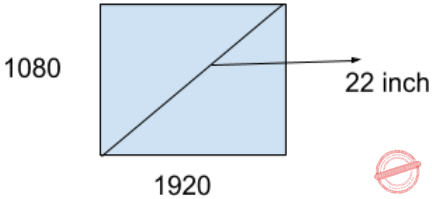Step-1: To check the ratio [width/height= 1920 /1080 ]
Step-2: Ratio: 16/9.
Step-3: Using Pythagoras theorem (9x)​ 2​ +(16x)​ 2​ =(22)​ 2
x=19.17 inch
 Question 16
Consider the following statements:
a) 3D Studio Max includes a number of high-level professional tools for character animation, game development, and visual effect production.
b) MAYA is a complete modeling package developed by Microsoft
c) RenderMan is a rendering package developed by Pixar
Which of the above statements are true?
 A Only a and b B Only a and c C Only b and c D a,b and c
Computer-Graphics       3D       KVS 30-12-2018 Part B
Question 16 Explanation:
MAYA 3D animation software offers a comprehensive creative feature set for 3D computer animation, modeling, simulation, rendering, and compositing on a highly extensible production platform. Maya has next-generation display technology, accelerated modeling workflows, and tools for handling complex data.
 Question 17
The ____ file format allows storing an animation sequence
 A PNG B GIF C JPG D PDF
Computer-Graphics       Motion-Pictures       KVS 30-12-2018 Part B
Question 17 Explanation:
→PDF means portable document format.
→Portable Network Graphics is a raster-graphics file-format that supports lossless data compression.
→JPG/JPEG: Joint Photographic Experts Group. JPEG is usually known as JPG. It stands for Joint Photographic Expert Group, a joint working group of International Standardization Organization (ISO) and International Electrotechnical Commission (IEC). It is a standard method of compressing graphic images.
→A GIF is a computer file that is used on the internet for sending images, especially moving images. GIF is an abbreviation for 'Graphic Interchange Format'.
 Question 18
​If the frame buffer has 10-bits per pixel and 8-bits are allocated for each of the R,G and B components then what would be the size of the color lookup table(LUT)
 A (2​ 10​ +2​ 11​ ) bytes B (2​ 10​ +2​ 8​ ) bytes C (2​ 10​ + 2​ 24​ ) bytes D (2​ 8​ + 2​ 9​ ) bytes
Computer-Graphics       LUT       UGC NET CS 2018-DEC Paper-2
Question 18 Explanation:
10-bits per pixel it means we will have 2​ 10 ​ entries in color lookup table.
8-bits are allocated for each of the R,G and B components, means each entry in color lookup table is of 24-bits( 8-bits for each of the R, G and B component).
24-bits = 3 Bytes
So the size of lookup table is = Number of entries * size of each entry
So the size of lookup table is = (2​ 10​ * 3) Bytes
the size of lookup table is = 3072 Bytes = (2​ 10​ + 2​ 11​ ) Bytes
 Question 19
Which homogeneous 2D matrix transforms the figure (a) on the left side to the figure (b) on the right ?AB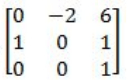CD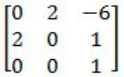Computer-Graphics       2-D-Geometrical-Transforms-and-Viewing       UGC NET CS 2018-DEC Paper-2
Question 19 Explanation:
The homogeneous coordinates of the cartesian point (x,y) is (x,y,1) and the transformation Matrix is a 3x3 Matrix.
The image in figure ​ a ​ is translated(by 1 unit in x axis), scaled( 2 units in y axis) and rotated(90 degrees counterclockwise) to figure in b.
 Question 20
​In 3D Graphics, which of the following statements about perspective and parallel projection is/are true?
P:​ In a perspective projection, the farthest an object is from the center of projection, the smaller it appears.
Q: ​ Parallel projection is equivalent to a perspective projection where the viewer is standing infinitely far away
R: ​ Perspective projections do not preserve straight lines.
 A P and R only B P,Q and R C Q and R only D P and Q only
Computer-Graphics       3D-Geomentry       UGC NET CS 2018-DEC Paper-2
Question 20 Explanation:
Perspective Projection​ :
Perspective projection is representing or drawing objects which resemble the real thing.
Perspective projection preserves the straight line.
In perspective projection, objects that are far away appear smaller, and objects that are near appear bigger.
Parallel lines do not remain parallel
Distance and angles are not preserved.
Parallel Projection :
In this projection drawing objects looks less realistic.
In this projection parallel lines remains parallel.
Angles are not preserved in this projection.
It is good for exact measurements.
 Question 21
​In 3D Graphics, which of the following statement/s is/are true ?
P: Back-face culling is an example of an image-precision visible-surface determination.
Q: Z-Buffer is a 16-bit, 32-bit, or 64-bit field associated with each pixel in a frame buffer that can be used to determine the visible surface at each pixel.
 A P only B Q only C Neither P nor Q D P and Q
Computer-Graphics       3D-Gepmetry       UGC NET CS 2018-DEC Paper-2
Question 21 Explanation:
Back Face Culling:
→ Back-face culling (an object space algorithm) works on 'solid' objects which you are looking at from the outside. That is, the polygons of the surface of the object completely enclose the object.
→ Back-face culling is not an example of an image-precision visible-surface determination.
→ Back-face culling can very quickly remove unnecessary polygons. Unfortunately there are often times when back-face culling can not be used. For example if you wish to make an open-topped box - the inside and the outside of the box both need to be visible, so either two sets of polygons must be generated, one set facing out and another facing in, or back-face culling must be turned off to draw that object.
1. Back faces: faces of opaque object which are “pointing away” from viewer
2. Back face culling – remove back faces (supported by OpenGL)
→ TRUE: Z-Buffer is a 16-bit, 32-bit, or 64-bit field associated with each pixel in a frame buffer that can be used to determine the visible surface at each pixel.
 Question 22
Which API is used to draw a circle ?
 A Circle( ) B Ellipse( ) C Round Rect( ) D Pie( )
Computer-Graphics       API       UGC NET CS 2012 Dec-Paper-2
Question 22 Explanation:
→ When we are giving same height and width to a ellipse it will become circle.
→ API is a protocol intended to be used as an interface by software components to communicate with each other.
→ An API is a library that may include specification for routines, data structures, object classes and variables
 Question 23
The LED’s for their display require
 A A voltage of 1.2v and a current of 20 mA B A voltage of 20v and a current of 1.2 A C A voltage of 1.2v and current of 100 mA D A voltage of 10v and a current of 120 mA
Computer-Graphics       LED       NIELIT Technical Assistant_2016_march
 Question 24
Two equal voltages os same frequency applied to the X and Y plates of a CRD, produces a circle on the screen. The phase difference between the two voltages is
 A 30o B 90o C 180o D 0o
Computer-Graphics       CRD       NIELIT Technical Assistant_2016_march
 Question 25
The spacing between character pairs is called :
 A Kerning B x-height C intercap D serif
Computer-Graphics       Spacing       UGC NET CS 2009-June-Paper-2
Question 25 Explanation:
→ kerning is the process of adjusting the spacing between characters in a proportional font, usually to achieve a visually pleasing result.
→ Kerning adjusts the space between individual letter forms, while tracking (letter-spacing) adjusts spacing uniformly over a range of characters.
 Question 26
Which of the following is not a component of Memory tube display ?
 A Flooding gun B Collector C Ground D Liquid Crystal
Computer-Graphics       Display-System       UGC NET CS 2017 Nov- paper-3
Question 26 Explanation:
Memory tube display components:
1. Connector Pins
2. Electron Gun
3. Base
4. Focusing System
5. Control Grid Voltage
6. X/Y Deflect
7. Phosphor
8. Collector
9. Ground
Note: Liquid crystal is not a component of memory tube display
.
 Question 27
Which of the following is not true in case of Oblique Projections?
 A Parallel projection rays are not perpendicular to the viewing plane. B Parallel lines in space appear parallel on the final projected image. C Used exclusively for pictorial purposes rather than formal working drawings. D Projectors are always perpendicular to the plane of projection.
Computer-Graphics       Oblique-Projections       UGC NET CS 2017 Nov- paper-3
 Question 28
With respect to CRT, the horizontal retrace is defined as:
 A The path an electron beam takes when returning to the left side of the CRT. B The path an electron beam takes when returning to the right side of the CRT. C The technique of turning the electron beam off while retracing. D The technique of turning the electron beam on/off while retracing.
Computer-Graphics       Display-System       UGC NET CS 2017 Nov- paper-3
 Question 29
A 4*4 DFT matrix is given by :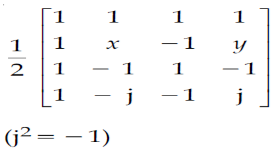Where values of x and y are _____, _____ respectively.
 A 1, −1 B −1, 1 C −j, j D j, −j
Computer-Graphics       DFT-Matrix       UGC NET CS 2017 Nov- paper-3
 Question 30
Which of the following statement(s) is/are correct ?
 A Persistence is the term used to describe the duration of phosphorescence. B The control electrode is used to turn the electron beam on and off. C The electron gun creates a source of electrons which are focused into a narrow beam directed at the face of CRT. D All of the above
Computer-Graphics       Display-System       UGC NET CS 2017 Jan- paper-3
Question 30 Explanation:
TRUE: Persistence is the term used to describe the duration of phosphorescence. Phosphorescence is a process in which energy absorbed by a substance is released relatively slowly in the form of light.
TRUE: The control electrode is used to turn the electron beam on and off.
TRUE: The electron gun creates a source of electrons which are focused into a narrow beam directed at the face of CRT.
 Question 31
A segment is any object described by GKS commands and data that start with CREATE SEGMENT and Terminates with CLOSE SEGMENT command. What functions can be performed on these segments ?
 A Translation and Rotation B Panning and Zooming C Scaling and Shearing D Translation, Rotation, Panning and Zooming
Computer-Graphics       Transformations       UGC NET CS 2017 Jan- paper-3
 Question 32
Match the following:A a-i, b-ii, c-iii, d-i B a-i, b-iii, c-ii, d-iv C a-iv, b-iii, c-ii, d-i D a-iv, b-ii, c-i, d-iii
Computer-Graphics       Display-System       UGC NET CS 2017 Jan- paper-3
 Question 33
Below are the few steps given for scan-converting a circle using Bresenham’s Algorithm. Which of the given steps is not correct ?
 A Compute d = 3 – 2r (where r is radius) B Stop if x > y C If d < 0, then d = 4x + 6 and x = x + 1 D If d ≥, then d = 4 * (x – y) + 10, x = x + 1 and y = y + 1
Computer-Graphics       Bresenham’s-Algorithm       UGC NET CS 2017 Jan- paper-3
Question 33 Explanation:
Scan converting a circle using Bresenham’s algorithm:
1 Initially X = 0 , Y = R and D = 3 – 2R

2. While (X < Y)

3. Call Draw Circle(Xc, Yc, X, Y)

4. Set X = X + 1

5. If (D < 0) Then

6. D = D + 4X + 6

7. Else

8. Set Y = Y – 1

9. D = D + 4(X – Y) + 10

10. End If

11. Draw Circle(Xc, Yc, X, Y) /* calling function call */

12. End While
 Question 34
Which of the following is/are side effects of scan conversion ?
a. Aliasing
b. Unequal intensity of diagonal lines
c. Overstriking in photographic applications
d. Local or Global aliasing
 A a and b B a, b and c C a, c and d D a, b, c and d
Computer-Graphics       Aliasing       UGC NET CS 2017 Jan- paper-3
Question 34 Explanation:
Side effects of scan conversions are 1. Aliasing
2. Unequal intensity of diagonal lines
3. Overstriking in photographic applications
4. Local or Global aliasing
 Question 35
Consider a line AB with A=(0,0) and B=(8,4). Apply a simple DDA algorithm and compute the first four plots on this line.
 A [(0, 0), (1, 1), (2, 1), (3, 2)] B [(0, 0), (1, 1.5), (2, 2), (3, 3)] C [(0, 0), (1, 1), (2. 2.5), (3, 3)] D [(0, 0), (1, 2), (2, 2), (3, 2)]
Computer-Graphics       DDA-Algorithm       UGC NET CS 2017 Jan- paper-3
Question 35 Explanation:Question 36
Consider the Breshenham’s circle generation algorithm for plotting a circle with centre (0, 0) and radius ‘r’ units in first quadrant. If the current point is (xi , yi ) and decision parameter is pi then what will be the next point (xi + 1, yi + 1 + 1) and updated decision parameter pi + 1 for pi ≥ 0?
 A xi + 1 = xi+ 1 yi + 1 = yi pi + 1 = pi + 4xi + 6 B xi + 1 = xi + 1 yi + 1 = yi - 1 pi + 1 = pi + 4(xi - yi) + 10 C xi + 1 = xi yi + 1 = yi - 1 pi + 1 = pi + 4(xi - yi) + 6 D xi + 1 = xi - 1 yi + 1 = yi pi + 1 = pi + 4(xi - yi) + 10
Computer-Graphics       Bresenham’s-Algorithm       UGC NET CS 2016 July- paper-3
Question 36 Explanation:
Write the steps required to scan - convert a circle using Bresenham’s algorithm.
Set the initial values of the variables: (h, k) = coordinates of circle center; x=0; y=circle radius r and d = 3 - 2r.
Test to determine whether the entire circle has been scan-converted. If x>y, stop.
Plot the eight points, found by symmetry with respect to the center (h, k), at the current (x, y) coordinates:
Plot(x+h, y+k) Plot(-x+h, -y+k)
Plot(y+h, x+k) Plot(-y+h, -x+k)
Plot(-y+h, x+k) Plot(y+h, -x+k)
Plot(-x+h, y+k) Plot(x+h, -y+k)
Compute the location of the next pixel. If d<0, then d=d+4x+6 and x=x+1. If d≥0, then d=d+4(x-y)+10, x=x+1 and y=y-1.
Go to step 2.
 Question 37
A point P(5, 1) is rotated by 90° about a pivot point (2, 2). What is the coordinate of new transformed point P′ ?
 A (3, 5) B (5, 3) C (2, 4) D (1, 5)
Computer-Graphics       Transformations       UGC NET CS 2016 July- paper-3
Question 37 Explanation:
Rotation around a pivot point (2, 2) can be represented as:
(h, k) = (2, 2)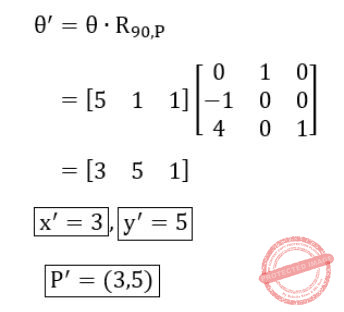Question 38
Let R be the rectangular window against which the lines are to be clipped using 2D Sutherland-Cohen line clipping algorithm. The rectangular window has a lower left-hand corner at (– 5, 1) and upper right-hand corner at (3, 7). Consider the following three lines for clipping with the given endpoint coordinates:
Line AB : A (– 6, 2) and B (–1, 8)
Line CD : C (– 1, 5) and D (4, 8)
Line EF : E (–2, 3) and F (1, 2)
Which of the following line(s) is/are candidate for clipping?
 A AB B CD C EF D AB and CD
Computer-Graphics       Sutherland-Cohen-algorithm       UGC NET CS 2016 July- paper-3
 Question 39
In perspective projection, if a line segment joining a point which lies in front of the viewer to a point in back of the viewer is projected to a broken line of infinite extent. This is known as _______.
 A View confusion B Vanishing point C Topological distortion D Perspective foreshortening
Computer-Graphics       Topological-Distortion       UGC NET CS 2016 July- paper-3
 Question 40
Let us consider that the original point is (x, y) and new transformed point is (x′, y′). Further, Shx and Shy are shearing factors in the x and y directions. If we perform the y-direction shear relative to x = xref then the transformed point is given by _______.
 A x′ = x + Shx ⋅ (y – yref) y′ = y B x′ = x y′ = y ⋅ Shx C x′ = x y′ = Shy (x – xref) + y D x′ = Shy ⋅ y y′ = y ⋅ (x – xref)
Computer-Graphics       Transformations       UGC NET CS 2016 July- paper-3
Question 40 Explanation:Question 41
Which of the following statement(s) is/are correct with reference to curve generation?
I. Hermite curves are generated using the concepts of interpolation.
II. Bezier curves are generated using the concepts of approximation.
III. The Bezier curve lies entirely within the convex hull of its control points.
IV. The degree of Bezier curve does not depend on the number of control points.
 A I, II and IV only B II and III only C I and II only D I, II and III only
Computer-Graphics       Bezier -Curves       UGC NET CS 2016 July- paper-3
 Question 42
A triangulation of a polygon is a set of T chords that divide the polygon into disjoint triangles. Every triangulation of n-vertex convex polygon has _____ chords and divides the polygon into _____ triangles.
 A n – 2, n – 1 B n – 3, n – 2 C n – 1, n D n – 2, n – 2
Computer-Graphics       Polygon       UGC NET CS 2016 July- paper-3
 Question 43
Consider a raster grid having XY-axes in positive X-direction and positive upward Y-direction with Xmax = 10, Xmin = –5, Ymax = 11, and Ymin = 6. What is the address of memory pixel with location (5, 4) in raster grid assuming base address 1 (one) ?
 A 150 B 151 C 160 D 161
Computer-Graphics       Raster-Systems       UGC NET CS 2016 Aug- paper-3
 Question 44
Consider a N-bit plane frame buffer with W-bit wide lookup table with W > N. How many intensity levels are available at a time ?
 A 2N B 2 W C 2 N+W D 2 N-1
Computer-Graphics       Frame-Buffer       UGC NET CS 2016 Aug- paper-3
 Question 45
Consider the Bresenham's line generation algorithm for a line with gradient greater than one, current point (xi, yi) and decision parameter, di. The next point to be plotted (xi+1, yi+1) and updated decision parameter, di+1, for di < 0 are given as _______.
 A xi+1 = xi +1 yi+1 = yi di+1 = di+ 2 dy B xi+1 = xi yi+1 = yi +1 di+1 = di+ 2 dx C xi+1 = xi yi+1 = yi +1 di+1 = di+ 2(dx -dy) D xi+1 = xi +1 yi+1 = yi +1 di+1 = di+ 2(dy -dx)
Computer-Graphics       Bresenham’s-Algorithm       UGC NET CS 2016 Aug- paper-3
Question 45 Explanation: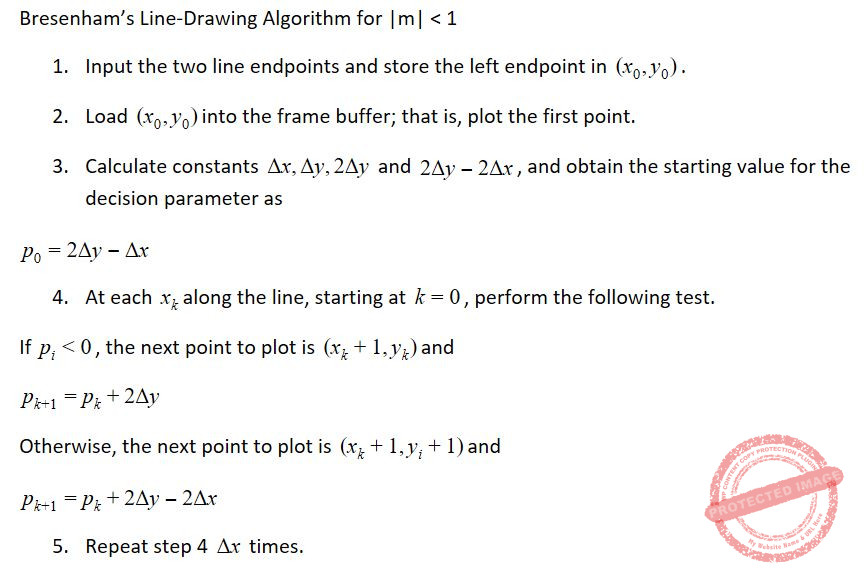Question 46
A point P(2, 5) is rotated about a pivot point (1, 2) by 60°. What is the new transformed point P' ?
 A (1, 4) B (–1, 4) C (1, – 4) D (– 4, 1)
Computer-Graphics       Transformations       UGC NET CS 2016 Aug- paper-3
Question 46 Explanation: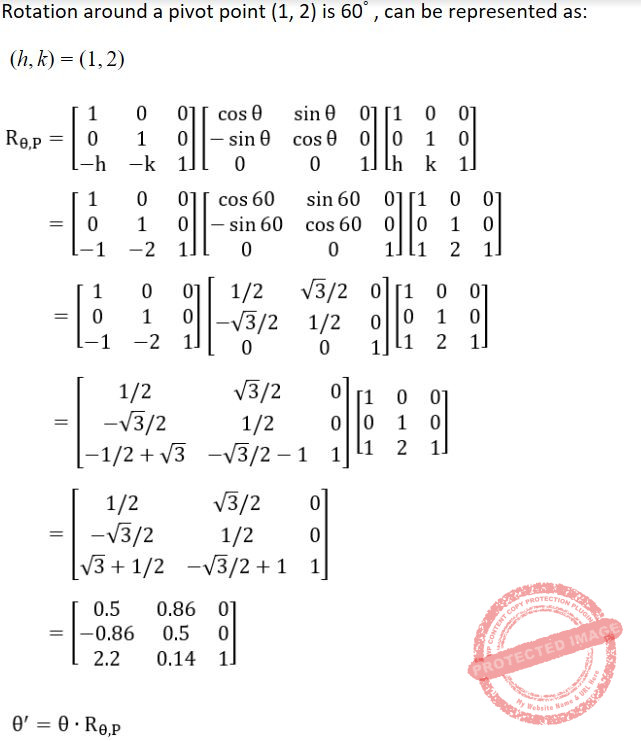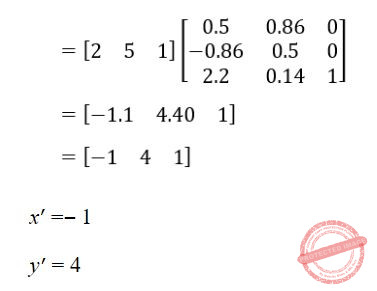Question 47
In perspective projection (from 3D to 2D), objects behind the centre of projection are projected upside down and backward onto the view-plane. This is known as _____.
 A Topological distortion B Vanishing point C View confusion D Perspective foreshortening
Computer-Graphics       3D       UGC NET CS 2016 Aug- paper-3
 Question 48
The Liang-Barsky line clipping algorithm uses the parametric equation of a line from (x1, y1) to (x2, y2) along with its infinite extension which is given as :
x = x1 + ∆x.u
y = y1 + ∆y.u
Where ∆x = x2– x1, ∆y = y2– y1, and u is the parameter with 0 ≤ u ≤ 1. A line AB with endpoints A(–1, 7) and B(11, 1) is to be clipped against a rectangular window with xmin = 1, xmax = 9, ymin = 2, and ymax = 8.
The lower and upper bound values of the parameter u for the clipped line using Liang-Barsky algorithm is given as :
 A (0, 2/3) B (1/6 , 5/6) C (0, 1/3) D (0, 1)
Computer-Graphics       Liang-Barsky-line-clipping-algorithm       UGC NET CS 2016 Aug- paper-3
 Question 49
Which of the following graphic primitives are considered as the basic building blocks of computer graphics ?
(a)Points
(b)Lines
(c)Polylines
(d)Polygons
 A (a) only B (a) and (b) C (a), (b) and (c) D (a), (b), (c) and (d)
Computer-Graphics       Basic-Building-Blocks       UGC NET CS 2015 Dec - paper-3
Question 49 Explanation:
Ponits, lines and polygon lines are the basic building blocks of computer graphics
 Question 50
Which of the following is/are the principal components of a memory-tube display ?
(a)Flooding gun
(b)Collector
(c)Phosphorus grains
(d)Ground
 A (a) and (b) B (c) only C (d) only D All the above
Computer-Graphics       Display-System       UGC NET CS 2015 Dec - paper-3
Question 50 Explanation:Question 51
Which raster locations would be chosen by Bresenham’s algorithm when scan converting a line from (1, 1) to (8, 5)?
 ABCDComputer-Graphics       Bresenham’s-Algorithm       UGC NET CS 2015 Dec - paper-3
Question 51 Explanation:Question 52
Consider a unit square centred at the origin. The coordinates of the square are translated by a factor (1/2, 1) and rotated by an angle of 90°. What shall be the coordinates of the new square?
 A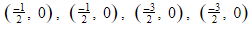BC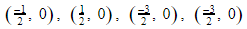DComputer-Graphics       Transformations       UGC NET CS 2015 Dec - paper-3
Question 52 Explanation: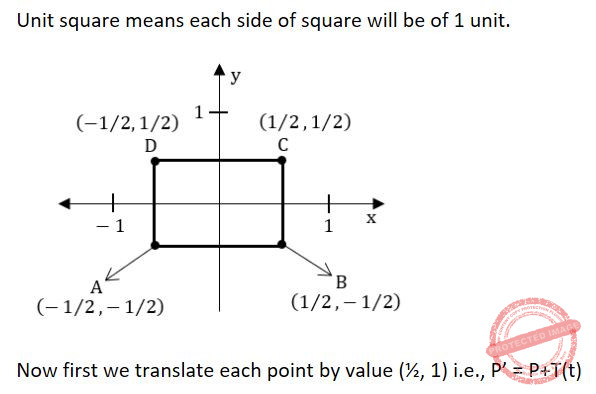Question 53
Which of the following is/are the components of a CRT?
(a)Electron Gun
(b)Control Electrode
(c)Focusing Electrode
(d)Phosphor Coated Screen
 A (a) and (d) B (a), (b) and (d) C (a), (b), (c) and (d) D (a), (c) and (d)
Computer-Graphics       Display-System       UGC NET CS 2015 Dec - paper-3
Question 53 Explanation: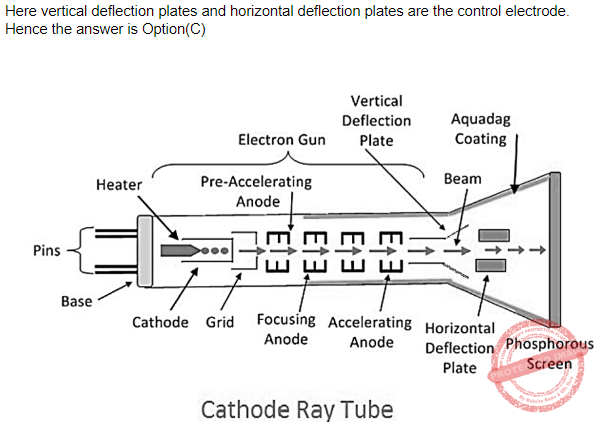Question 54
Using the phong reflectance model, the strength of the specular highlight is determined by the angle between
 A The view vector and the normal vector B The light vector and the normal vector C The light vector and the reflected vector D the reflected vector and the view vector
Computer-Graphics       Phong-Reflectance-Model       UGC NET June-2019 CS Paper-2
Question 54 Explanation:
Specular highlights on objects appear, because the light reflected from the object hits viewer. Sometimes, we are hit directly by the light and the highlight appears to be very intense, and sometimes we are hit so slightly, that we basically don't see the specular highlight. The strength of this highlight depends on the cosine of the angle between the reflected vector and vector taken from the point at object to the viewer's position: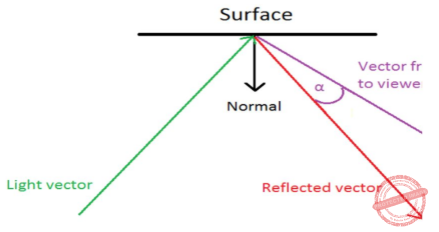Question 55
Give the number of principal vanishing point(s) along with their direction for the standard perspective transformation:
 A Only one in the direction K B Two in the directions I and J C Three in the directions I, J and K D Only two in the directions J and K
Computer-Graphics       Transformations       UGC NET CS 2015 June Paper-3
Question 55 Explanation:
There is only one principal vanishing point and it is in K-direction.
 Question 56A (a)-(i), (b)-(ii), (c)-(iii), (d)-(iv), (e)-(v) B (a)-(ii), (b)-(iii), (c)-(i), (d)-(iv), (e)-(v) C (a)-(iii), (b)-(i), (c)-(ii), (d)-(v), (e)-(iv) D (a)-(iv), (b)-(v), (c)-(i), (d)-(ii), (e)-(iii)
Computer-Graphics       Display-System       UGC NET CS 2015 June Paper-3
Question 56 Explanation:
Flood Gun: An electron gun designed to flood the entire screen with electrons.
Collector: Partly energized by flooding gun stores the charge generated by the writing gun.
Ground: Used to discharge the collector.
 Question 57
If we want to resize a 1024 × 768 pixels image to one that is 640 pixels wide with the same aspect ratio, what would be the height of the resized image?
 A 420 Pixels B 460 Pixels C 480 Pixels D 540 Pixels
Computer-Graphics       Aspect-Ratio       UGC-NET DEC-2019 Part-2
Question 57 Explanation:
Aspect Ratio= Width / Height
Aspect ration of 1024 × 768 pixels image = 1024/768
= 4/3
Aspect ration of modified pixels image = 640/height
4/3 = 640/height
Height = (3*640)/4
Height = 480 Pixels
 Question 58
Consider the following statement with respect to approaches to fill area on raster systems:
(P) To determine the overlap intervals for scan lines that cross the area.
(Q) To start from a given interior position and paint outward from this point until we encounter the specified boundary conditions.
Select the correct answer from the options given below:
 A P only B Q only C Both P and Q D Neither P nor Q
Computer-Graphics       Raster-Systems       UGC-NET DEC-2019 Part-2
Question 58 Explanation:
Fill area on raster systems:
TRUE: To determine the overlap intervals for scan lines that cross the area.
TRUE: To start from a given interior position and paint outward from this point until we encounter the specified boundary conditions.
 Question 59

Beam-penetration and shadow-mask are the two basic techniques for producing color displays with a CRT. Which of the following is not true ?

I. The beam-penetration is used with random scan monitors.

 A I and II B II and III C III only D IV only
Computer-Graphics       CRT       UGC NET CS 2014 June-paper-3
Question 59 Explanation:
Beam Penetration Method for displaying color pictures has been used with random-scan monitors. Two layers of phosphor, usually red and green, are coated on to the inside of the CRT screen, and the displayed color depends on how far the electron beam penetrates into the phosphor layers.
Shadow-mask Methods are commonly used in raster-scan systems (including color TV) because they produce a much wider range of color than the beam penetration method. A shadow-mask CRT has three phosphor color dots at each pixel position. One phosphor dot emits a red light, another emits a green light, and the third emits a blue light.
 Question 60
Line caps are used for adjusting the shape of the line ends to give them a better appearance. Various kinds of line caps used are
 A Butt cap and sharp cap B Butt cap and round cap C Butt cap, sharp cap and round cap D Butt cap, round cap and projecting square cap
Computer-Graphics       2D-Graphics       UGC NET CS 2014 June-paper-3
Question 60 Explanation:
Line caps helps in improving the appearance of the line by adjusting the shape of line ends There are three types of line caps:
1. But Cap 2. Round Cap 3. Projection Square Cap
 Question 61

Given below are certain output primitives and their associated attributes. Match each primitive with its corresponding attributes :

List – I List – II

a. Line i. Type, Size, Color

b. Fill Area ii. Color, Size, Font

c. Text iii. Style, Color, Pattern

d. Marker iv. Type, Width, Color
 A a-i, b-ii, c-iii, d-iv B a-ii, b-i, c-iii, d-iv C a-iv, b-iii, c-ii, d-i D a-iii, b-i, c-iv, d-ii
Computer-Graphics       2D-Graphics       UGC NET CS 2014 June-paper-3
Question 61 Explanation:
Line Attributes: Basic attributes of a straight line segment are its type, its width, and its color. In some graphics packages, lines can also be displayed using selected pen or brush options.
AREA-FILL ATTRIBUTES: Basic attributes of an area-fill are
Fill Styles: Areas are displayed with three basic fill styles: hollow with a color border, filled with a solid color, or Wed with a specified pattern or design.
Pattern Fill
Soft Fill: the linear soft-fill algorithm repaints an area that was originally painted by merging a foreground color F with a single background color 8, where F + B.The current RGB color P of each pixel within the area to be refilled is some linear combination of F and B:
P = tF + (1- t)B
where the "transparency" factor t has a value between 0 and 1 for each pixel. RGB component of the colors
P = (PR, PC, PR), F = (FR, Fc, FR), B = (BR, Bc, BB)
Text Attributes: Following are the text attributes:
Font : is the choice of font (or typeface), which is a set of characters with a particular design style such as New York, Courier, Helvetica, London, 'Times Roman, and various special symbol groups.
Font size
Font colour
Marker Attributes: A marker symbol is a single character that can be displayed in different colors and in different sizes. We select a particular character to be the marker symbol with
setMarkerType (int)
Hence Marker attributes are type, size and color.
 Question 62
Consider a window bounded by the lines : x = 0; y= 0; x = 5 and y = 3. The line segment joining (–1, 0) and (4, 5), if clipped against this window will connect the points
 A (0, 1) and (2, 3) B (0, 1) and (3, 3) C (0, 1) and (4, 3) D (0, 1) and (3, 2)
Computer-Graphics       Line-clipping       UGC NET CS 2014 June-paper-3
Question 62 Explanation:Question 63
Which of the following color models are defined with three primary colors ?
 A RGB and HSV color models B CMY and HSV color models C HSV and HLS color models D RGB and CMY color models
Computer-Graphics       Basics       UGC NET CS 2014 June-paper-3
Question 63 Explanation:
Common color models defined with three primary colors are the RGB and CMY models.
RGB: color scheme is an additive model. Intensities of the primary colors are added to produce other colors. Each color point within the bounds of the cube can be represented as a triple (R, G, B), where values for R, G, and B are assigned in the range from 0 to 1. Thus, a color C, is expressed in RGB components as
C = RR + GG + BB
CMY: A color model defined with the primary colors cyan, magenta, and yellow (CMY) is useful for describing color output to hard-copy devices. We can express the conversion from an RGB representation to a CMY representation with the matrix transformation:Question 64
A technique used to approximate halftones without reducing spatial resolution is known as _________.
 A Halftoning B Dithering C Error diffusion D None of the above
Computer-Graphics       Dithering       UGC NET CS 2014 Dec - paper-3
 Question 65

In Cyrus-Beck algorithm for line clipping the value of t parameter is computed by the relation :

(Here P1 and P2 are the two end points of the line, f is a point on the boundary, n1 is inner normal)
 A (P1 – fi) × ni / (P2 – P1) × ni B (fi – P1) × ni / (P2 – P1) × ni C (P2 – fi) × ni / (P1 – P2) × ni D (fi – P2) × ni / (P1 – P2) × ni
Computer-Graphics       Cyrus-Beck-Algorithm       UGC NET CS 2014 Dec - paper-3
 Question 66A a-i, b-iii, c-iv, d-ii B a-ii, b-iii, c-i, d-iv C a-iv, b-ii, c-iii, d-i D a-i, b-ii, c-iii, d-iv
Computer-Graphics       Projections       UGC NET CS 2014 Dec - paper-3
Question 66 Explanation:
Cavalier Projection: The direction of projection is chosen so that there is no foreshortening of lines perpendicular to the xy plane.
Cabinet Projection: The direction of projection is chosen so that lines perpendicular to the xy planes are foreshortened by half their lengths.
Isometric Projection: The direction of projection makes equal angles with all of the principal axis.
Orthographic Projection: Projections are characterized by the fact that the direction of projection is perpendicular to the view plane.
 Question 67
Concerning phong shading and gouraud shading in a 3D scene, which of the following statements are true? (A) Gouraud shading requires more computation than phong shading (B) Gouraud shading linearly interpolates the color of an interior pixel from the color at the vertices. (C) Phong shading interpolates over the normal vectors specified at the vertices. Choose the correct answer from the options given below:
 A (A) and (B) only B (A) and (C) only C (B) and (C) only D (A), (B) and (C)
Computer-Graphics       3D       UGC NET JRF November 2020 Paper-2
 Question 68
Given below are different properties of 3D projections from A.D. Identify the correct order on the basis of property true of (i) a perspective projection only (ii) an orthographic projection only (iii) both orthographic and projective transformations and (iv) neither orthographic nor projective transformation, respectively. (A) Straight lines are mapped to straight lines. (B) Distance and angles are (in general) preserved. (C) Far away objects appear the same size as closer ones. (D) Requires homogeneous coordinates in order for it to be encoded into linear transformation. Choose the correct answer from the options given below:
 A D, C, B, A B B, C, D, A C D, C, A, B D C, D, B, A
Computer-Graphics       Projections       UGC NET JRF November 2020 Paper-2
 Question 69
Match List-I with List-II. List-I gives 33 matrices representing 2D transformations and List-II shows the corresponding transformation diagrams.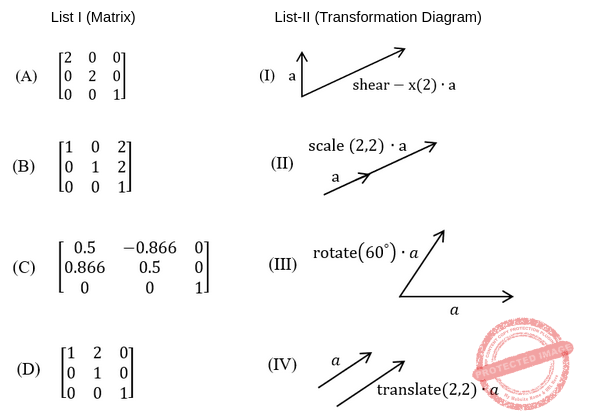Choose the correct answer from the options given below:
 A A-IV, B-II, C-III, D-I B A-IV, B-III, C-II, D-I C A-III, B-II, C-IV, D-I D A-II, B-IV, C-III, D-I
Computer-Graphics       Transformations       UGC NET JRF November 2020 Paper-2
 Question 70
Given below are two statements: Statement I: Bezier curves are curves that interpolate all of their control points Statement II: A cubic bezier curve has four control points. In the light of the above statements, choose the correct answer from the options given below
 A Both statement I and Statement II are true B Both Statement I and Statement II are false C Statement I is correct but Statement II is false D Statement I is incorrect but Statement II is true
Computer-Graphics       Bezier -Curves       UGC NET JRF November 2020 Paper-2
 Question 71
n the context of 3D Computer graphics, which of the following statements is/are correct? A) Under perspective projection, each set of parallel lines in the object do not stay parallel in the image (except those that are parallel to the view plane to start with). B) Applying a perspective transformation in the graphics pipeline to a vertex involves dividing by its ‘z’ coordinate. C) Perspective transformation is a linear transformation. Choose the correct answer from the options given below:
 A (A) and (B) only B (A) and (C) only C (B) and (C) only D (A), (B) and (C)
Computer-Graphics       3D       UGC NET JRF November 2020 Paper-2
There are 71 questions to complete.